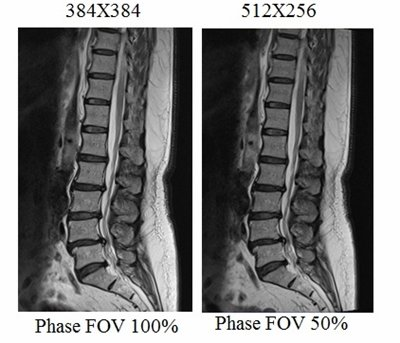mrimaster# MRI Resolution and Image Quality

### Introduction

Resolution refers to the human eye’s ability to distinguish between different structures. In MRI, resolution is determined by the number of pixels within a specified FOV (Field of View). The higher the image resolution, the more effective the diagnosis of small pathologies becomes. Resolution is directly proportional to the number of pixels – a greater pixel count leads to higher resolution. Pixel size can be calculated by dividing the field of view by the matrix size (e.g., FOV 320, Matrix 320×320, Pixel size = 320/320 = 1mm). MRI employs two resolution parameters for generating two-dimensional images: basic resolution and phase resolution.

### Basic resolution

Basic resolution is the number of pixels in the readout direction. It also determines the size of the image matrix. Basic resolution is inversely proportional to the size of the pixel, with lower resolution resulting in a higher pixel size.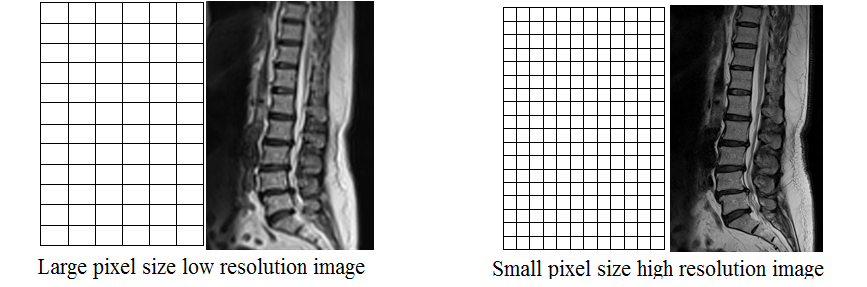### SNR and Basic resolution

The signal-to-noise ratio (SNR) is inversely proportional to the basic resolution. In other words, SNR is directly proportional to the pixel size. Increasing the base resolution will decrease the pixel size, consequently reducing the SNR of the image.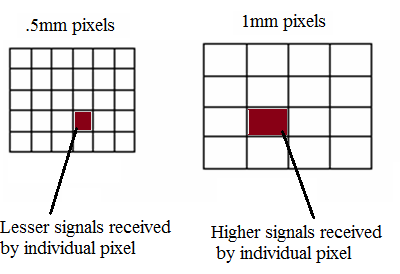When comparing a 256 x 256 matrix format with a 128 x 128 matrix format, the pixel size of the 128 matrix (2x2mm) is four times larger than that of the 256 matrix (1x1mm). However, there are only half the phase encoding steps (lines in k-space) in the 128 matrix. Therefore, the Signal-to-Noise Ratio (SNR) is calculated as approximately 4/√2 ≈ 2.82.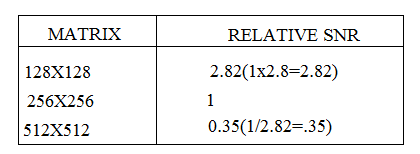Increasing the basic resolution will improve the image quality. However, going beyond the acceptable range of resolution will introduce graininess to the image due to low SNR. Conversely, reducing it below the acceptable range will result in a blurry image due to high SNR. Furthermore, increasing the basic resolution will lead to a longer scan time.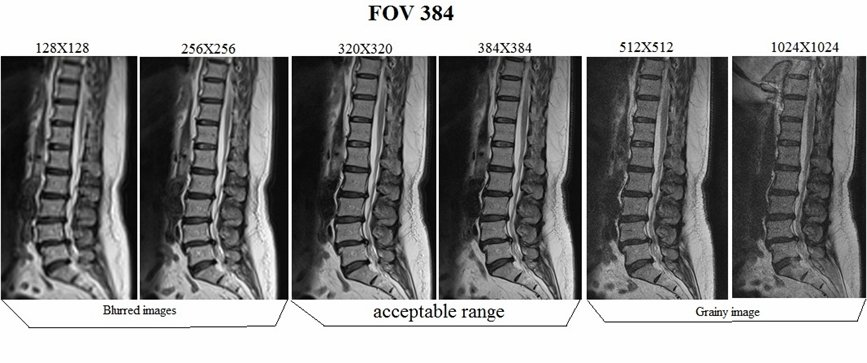### How to manipulate a grainy image?

Reduce the base resolution by one or two steps. Reducing the base resolution will reduce the scan time.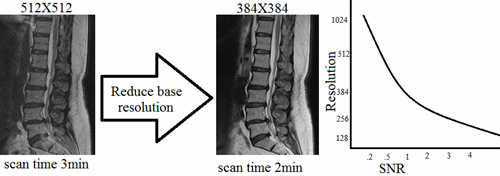Increase slices thickness. Will increase the voxel size and SNR there for the image will become smoother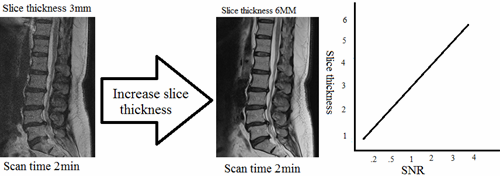Decrease bandwidth. Decreasing bandwidth will increase the TR and TE therefore it will increase the scan time.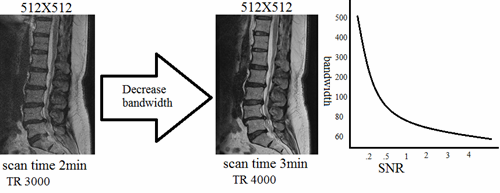Increase FOV. Increasing FOV increase the pixel size and SNR therefore the image will become smoother.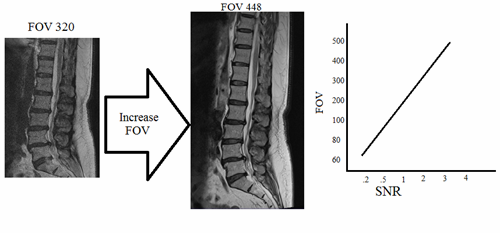Increase average (NSA).increasing NSA will increase the scan time.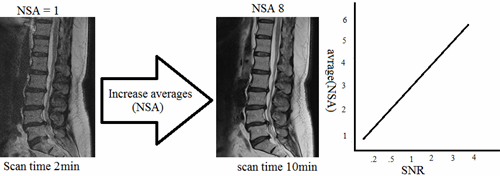### How to manipulate a low resolution blurred image?

Increase the base resolution by one or two steps. Increasing base resolution will prolong the scan time.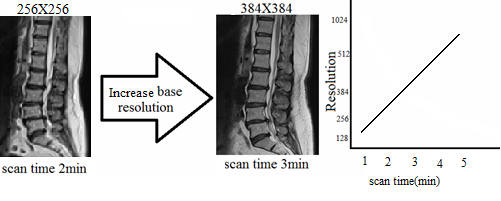Decrease FOV. Decreasing FOV reduces the pixel size and SNR therefore the image will become sharper.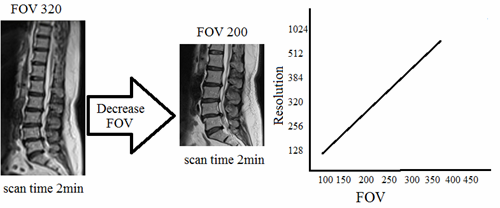### SNR and Basic resolution

Phase resolution is the number of pixels in the phase direction. It is normally expressed as a percentage of the basic resolution. Decreasing the phase resolution will increase the pixel size in one direction and result in a rectangular pixel shape.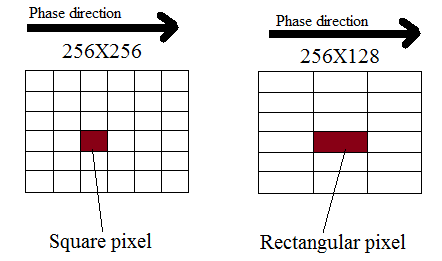Decreasing the phase resolution will reduce image quality and scan time. Reducing the phase resolution will increase pixel size; therefore, the signal-to-noise ratio (SNR) will increase considerably.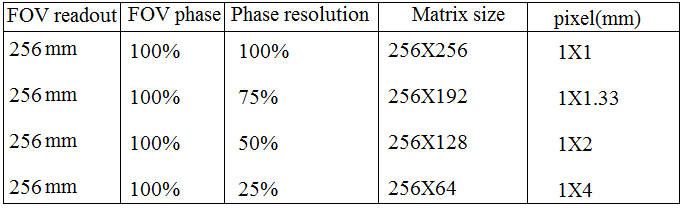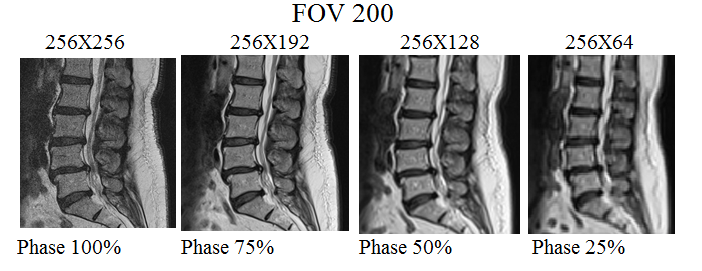In addition to the basic resolution, image quality can be manipulated by changing the phase resolution. Similar image quality can be obtained from 384×384 matrices and 512×256 matrices.